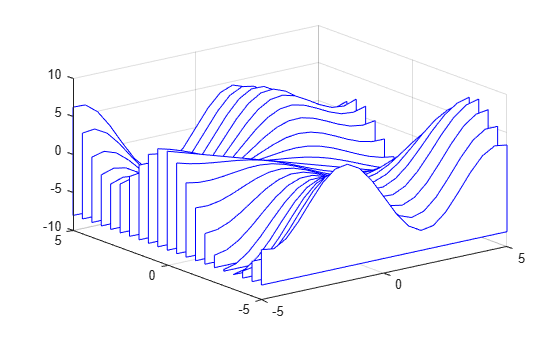waterfall

Waterfall plot

Description

example

waterfall(X,Y,Z) creates a waterfall plot, which is a mesh plot with a partial curtain along the y dimension. This results in a "waterfall" effect. The function plots the values in matrix Z as heights above a grid in the xy-plane defined by X and Y. The edge colors vary according to the heights specified by Z.

example

waterfall(X,Y,Z,C) additionally specifies the color of the edges.

waterfall(Z) creates a waterfall plot, and uses the column and row indices of the elements in Z as the x- and y-coordinates.

waterfall(Z,C) additionally specifies the color of the edges.

waterfall(ax,___) plots into the axes specified by ax instead of the current axes. Specify the axes as the first input argument. This argument can be used with any of the previous input syntaxes.

example

p = waterfall(___) returns the patch object. Use p to modify the waterfall plot after it is created. For a list of properties, see Patch Properties.

Examples

collapse all

Create three matrices of the same size. Then plot them as a waterfall plot. The mesh plot uses Z for both height and color.

[X,Y] = meshgrid(-3:.125:3);
Z = peaks(X,Y);
waterfall(X,Y,Z)Specify the colors for a waterfall plot by including a fourth matrix input, C. The waterfall plot uses Z for height and C for color. Add a color bar to the graph to show how the data values in C correspond to the colors in the colormap.

[X,Y] = meshgrid(-3:.125:3);
Z = peaks(X,Y);
waterfall(X,Y,Z,C)
colorbarCreate a waterfall plot. To allow further modifications, assign the patch object to the variable p.

[X,Y] = meshgrid(-5:.5:5);
Z = Y.*sin(X) - X.*cos(Y);
p = waterfall(X,Y,Z)p =
Patch with properties:

FaceColor: [1 1 1]
FaceAlpha: 1
EdgeColor: 'flat'
LineStyle: '-'
Faces: [21x26 double]
Vertices: [546x3 double]

Show all properties

Use p to access and modify properties of the waterfall plot after it is created. For example, change the color of the plot edges by setting the EdgeColor property.

p.EdgeColor = 'b';Display a partial curtain along the x-dimension (instead of the y-dimension) by transposing the input arguments.

[X,Y] = meshgrid(-3:.125:3);
Z = peaks(X,Y);
waterfall(X',Y',Z')Input Arguments

collapse all

x-coordinates, specified as a matrix the same size as Z, or as a vector with length n when Z is an m-by-n matrix. If you do not specify values for X and Y, waterfall uses the vectors (1:n) and (1:m).

The XData property of the patch object stores the x-coordinates.

Example: X = 1:10

Example: X = [1 2 3; 1 2 3; 1 2 3]

Example: [X,Y] = meshgrid(-5:0.5:5)

Data Types: single | double | int8 | int16 | int32 | int64 | uint8 | uint16 | uint32 | uint64 | categorical

y-coordinates, specified as a matrix the same size as Z or as a vector with length m when Z is an m-by-n matrix. If you do not specify values for X and Y, waterfall uses the vectors (1:n) and (1:m).

The YData property of the patch object stores the y-coordinates.

Example: Y = 1:10

Example: Y = [1 1 1; 2 2 2; 3 3 3]

Example: [X,Y] = meshgrid(-5:0.5:5)

Data Types: single | double | int8 | int16 | int32 | int64 | uint8 | uint16 | uint32 | uint64 | categorical

z-coordinates, specified as a matrix. Z must have at least two rows and two columns.

Z specifies the height of the waterfall plot at each xy-coordinate. If you do not specify the colors, then Z also specifies the plot edge colors.

The ZData property of the patch object stores the z-coordinates.

Example: Z = [1 2 3; 4 5 6]

Example: Z = sin(x) + cos(y)

Data Types: single | double | int8 | int16 | int32 | int64 | uint8 | uint16 | uint32 | uint64 | categorical

Color indexing array, specified as an m-by-n matrix of colormap indices, where Z is m-by-n. For each grid point on the waterfall plot, C indicates a color in the colormap. The CDataMapping property of the patch object controls how the values in C correspond to colors in the colormap.

The CData property of the patch object stores the color array. For additional control over the plot coloring, use the FaceColor and EdgeColor properties.

Target axes, specified as an Axes object. If you do not specify the axes, then waterfall plots into the current axes.

Tips

• To analyze the data as columns instead of rows, call waterfall with transposed arguments:

[X,Y] = meshgrid(-3:.125:3);
Z = peaks(X,Y);
waterfall(X',Y',Z')

• To create a mesh surface object instead of a patch object, use the meshz function. To create a plot similar to a waterfall plot, set the MeshStyle property of the surface to 'Row'.

Algorithms

The range of X, Y, and Z, or the current setting of the axes' XLim, YLim, and ZLim properties, determines the range of the axes (also set by axis). The range of C, or the current setting of the axes' CLim property, determines the color scaling (also set by caxis).